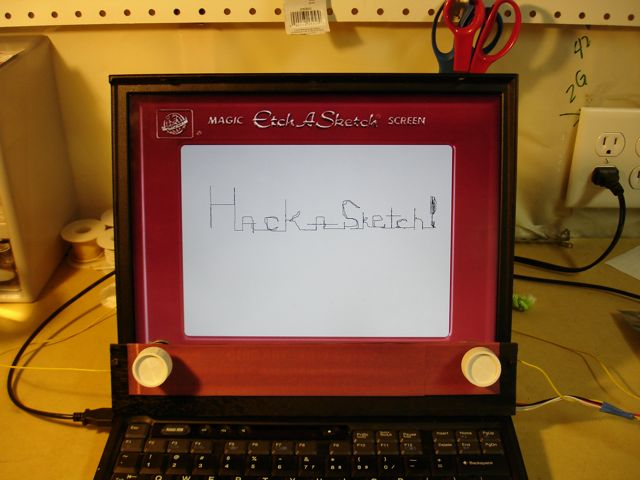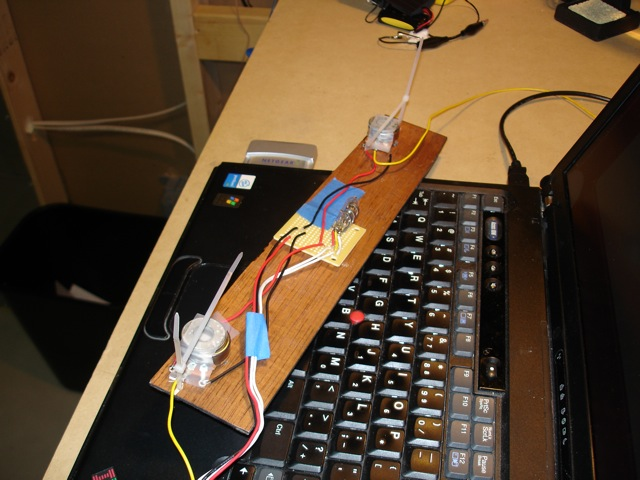Select Page

Difficulty Level = 5 [What’s this?]

Here is a device I call the Hack-a-Sketch. The screen is a normal laptop (an old one), but it has real knobs which control the stylus on the screen.The Hack-a-Sketch

An Arduino board reads the inputs from two potentiometers (the knobs), and sends the information via USB to a Processing sketch which displays the path of the stylus on the screen. This was extremely easy to build because the Arduino is just running the StandardFirmata firmware. No custom code on the board. The Processing sketch was surprisingly easy to write. Using this really did feel like using an Etch-a-Sketch.

Here’s the Hack-a-Sketch in action. Wait for the big finish where I erase the image…

How did I erase the drawing by shaking the computer? There’s a mercury switch hidden behind the panel holding the knobs. When the code senses shaking, the image is slowly erased. More shaking = more erasure.Disassembled Hack-a-Sketch exposing mercury switch to sense movement.

Finally, here’s the Processing code that does all the work:

```import processing.serial.*;

import processing.core.*;
import cc.arduino.Arduino;

PImage backgroundImage;
Arduino arduino;
int STYLUS_X_PIN = 0;
int STYLUS_Y_PIN = 1;
int MERCURY_SWITCH_LEFT_PIN = 8;
int MERCURY_SWITCH_RIGHT_PIN = 9;
// Origin is lower left corner of screen.
int originX = 146;
int originY = 673;
int width = 740;
int height = 530;
int PEN_COLOR = 50;
int GRAY = 217;
int GRAY_ALPHA = 80;
int lastX, lastY;
int SMOOTH_BUFFER_SIZE = 2;
int[] smoothBufferX = new int[SMOOTH_BUFFER_SIZE];
int[] smoothBufferY = new int[SMOOTH_BUFFER_SIZE];
int smoothBufferXSum = 0;
int smoothBufferYSum = 0;
int LEFT = 0;
int RIGHT = 1;
int SHAKE_SPEED_THRESHOLD = 300;
int lastSwitchPosition;
int lastSwitchPositionChange;

void setup() {
size(1024, 768);
frameRate(24);
background(backgroundImage);

String[] ports = Arduino.list();
println(ports);
println(ports);
String port = ports;
try {
arduino = new Arduino(this, port, 115200);
arduino.pinMode(MERCURY_SWITCH_LEFT_PIN, Arduino.INPUT);
arduino.pinMode(MERCURY_SWITCH_RIGHT_PIN, Arduino.INPUT);
} catch (Exception e) {
println("Failed to find Arduino board");
}
stroke(PEN_COLOR);
}

void draw() {
smooth();
int x, y;
if (frameCount == 1) {
// Give the Arduino some time to initialize so we can get a good first
delay(2000);
for(int i=0;i < SMOOTH_BUFFER_SIZE;i++) {
smoothBufferX[i] = lastX;
smoothBufferXSum += lastX;
smoothBufferY[i] = lastY;
smoothBufferYSum += lastY;
}
point(originX+lastX, originY-lastY);
}

if ((x != lastX) || (y != lastY)) {
line(originX+lastX, originY-lastY, originX+x, originY-y);
}
lastX = x;
lastY = y;

if (shake()) {
erase();
}
}

boolean shake() {
boolean left = (arduino.digitalRead(MERCURY_SWITCH_LEFT_PIN) == Arduino.HIGH);
if (left && (lastSwitchPosition != LEFT)) {
lastSwitchPosition = LEFT;
if ((millis() - lastSwitchPositionChange) < SHAKE_SPEED_THRESHOLD) {
lastSwitchPositionChange = millis();
return true;
}
lastSwitchPositionChange = millis();
}
boolean right = (arduino.digitalRead(MERCURY_SWITCH_RIGHT_PIN) == Arduino.HIGH);
if (right && (lastSwitchPosition != RIGHT)) {
lastSwitchPosition = RIGHT;
if ((millis() - lastSwitchPositionChange) < SHAKE_SPEED_THRESHOLD) {
lastSwitchPositionChange = millis();
return true;
}
lastSwitchPositionChange = millis();
}
return false;
}

void keyPressed() {
if (key == ' ') {
erase();
}
}

void erase() {
fill(GRAY, GRAY_ALPHA);
noStroke();
rect(originX, originY-height, width+1, height+1);
stroke(PEN_COLOR);
}

// Read current stylus X position relative to the origin.
return (int)map((float)reading, 0f, 1023f, 0f, (float)width);
}

int smoothX(int v) {
int index = frameCount % SMOOTH_BUFFER_SIZE;
smoothBufferXSum -= smoothBufferX[index];
smoothBufferXSum += v;
smoothBufferX[index] = v;
return (int)(smoothBufferXSum / SMOOTH_BUFFER_SIZE);
}

// Read current stylus Y position relative to the origin.